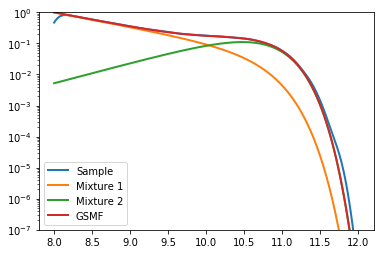# Construct a custom fit for an extension of the MRP: a double-Schechter function.¶

Note: this example is almost identical to that found in the R package tggd in the tggd_log documentation.

While mrpy has several in-built routines which aid in fitting the MRP function to data, it does not natively support fitting extensions of the MRP, such as double-Schechter functions. For such functions, one can fairly simply create custom fits using the methods found in Scipy, for example.

In this example, we create a double-Schechter galaxy stellar mass function (GSMF) down to a target stellar mass (xmin) of log10(SM) = 8. We use data from Baldry+2012 to define the function:

Both mixtures have

\begin{align}\begin{aligned}M_\star \equiv \log_{10} \mathcal{H}_\star = 10.66\\and\end{aligned}\end{align}
$\beta = 1$

Mixture 1 has:

$\alpha_1 = -1.47$
$\phi^\star_1 = 0.79\times 10^{-3}$

Mixture 2 has:

$\alpha_2 = -0.35$
$\phi^\star_2 = 3.96\times 10^{-3}$

Furthermore, we use only the purely statistical routines of mrpy to achieve our results:

In :

# Imports
%matplotlib inline
import matplotlib.pyplot as plt
import numpy as np
from scipy.stats import gaussian_kde
from scipy.optimize import minimize

from mrpy.base import stats


First we define objects which capture the statistical quantities of each mixture:

In :

mix1 = stats.TGGDlog(10.66,-1.47,1.0,8)
mix2 = stats.TGGDlog(10.66,-0.35,1.0,8)


$$\phi^\star$$ is defined as the value of the pdf in log-space at the pivot scale by $$e$$, i.e.

$\phi^\star = PDF(M_\star)\times \frac{e}{\ln(10)}$

This normalisation is important in our sampling since it gives the ratio of samples produced by each mixture. We can produce the relevant normalisation using:

In :

M1norm=0.79/mix1.pdf(10.66)
M2norm=3.96/mix2.pdf(10.66)
Mtot=M1norm+M2norm


Now say we would like to sample 1e5 galaxies, we can produce these like so:

In :

Nsamp=1e5

np.random.seed(100)

mix1_sample = mix1.rvs(int(Nsamp*M1norm/Mtot))
mix2_sample = mix2.rvs(int(Nsamp*M2norm/Mtot))

gal_sample = np.concatenate((mix1_sample,mix2_sample))


Let’s plot the distribution we’ve just created, to check if everything has worked okay:

In :

# Create x array to plot against
x = np.linspace(8,12,401)

plt.plot(x,gaussian_kde(gal_sample)(x),label="Sample", lw=2)

plt.plot(x,mix1.pdf(x) * M1norm/Mtot,label="Mixture 1", lw=2)
plt.plot(x,mix2.pdf(x) * M2norm/Mtot,label="Mixture 2", lw=2)
plt.plot(x,mix1.pdf(x) * M1norm/Mtot + mix2.pdf(x) * M2norm/Mtot, label="GSMF", lw=2)
plt.yscale('log')
plt.ylim((1e-7,1))
plt.legend(loc=0)

Out:

<matplotlib.legend.Legend at 0x7fa87a9d5210>Now we can try to fit the mixed model. The trick here is we fit for the mixture using an additional parameter $$\lambda$$, where one component is multiplied by $$\lambda$$ and the other $$1-\lambda$$. We define it so that M1norm/Mtot=lambda and M1norm/Mtot=1-lambda. Here’s our model:

In :

def mix_lnl(par,data):
mix1 = stats.TGGDlog(par,par,1.0,8)
mix2 = stats.TGGDlog(par,par,1.0,8)

return -np.sum(np.log(mix1.pdf(data)*par + mix2.pdf(data)*(1-par)))


Now we can perform the fit, using a downhill-gradient method of our choice. The fit is probably not fantastic though. Generalised Gamma distributions (including truncated ones) display poor convergence properties using ML. Full MCMC is a better route when trying to fit GSMF type data. And the data certainly should not be binned!

In :

GSMFfit = minimize(mix_lnl, x0=[10,-2,0,0.5], args=(gal_sample,), bounds=[(9,12),(-2.5,-0.5),(-1.0,0.5),(0.0,1.0)])

print "Maximum likelihood parameters: ", GSMFfit.x

Maximum likelihood parameters:  [ 10.65427592  -1.46844631  -0.34935003   0.83445411]


This accords very well with our input parameters!